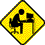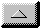Publications Department

Testing the Operation of a SETI System
by Using a Sun Traverse

by Ian Drummond VE6IXD
drummoni@enterprise.cybersurf.net
The method I used is described in two separate articles by David Shaffer W8MIF and Bob Atkins KA1GT in Chapter 7 of the ARRL UHF/Microwave Experimenter's Manual, published in 1990 by the American Radio Relay League, Newington, CT.

The basic concept is to measure the change in power output of the receiving system when pointed towards and away from the sun. As the solar flux at microwave frequencies arriving at the Earth is known, the sensitivity of the receiving system can be calculated.

The equipment I used:

• A 2.4 m square dish mounted on the original TV polar mount. It was not able to track the sun but could be manually rotated then left stationary, so the sun would pass across the pattern of the dish due to the rotation of the Earth.
• An Olde Antenna Lab helical feed connected directly by a male/male N-connector to a Downeast Microwave SETI-LNA preamplifier. The preamp was powered by a 12 volt lead-acid battery through a separate power line (not the coax cable).
• 20' of International 9096 coax connected the signal to an ICOM R-7100 receiver tuned to 1296 MHz in the USB mode.
The audio output of the receiver was fed to a simple envelope detector (see Figure 1 in the ARRL article), and the resulting DC voltage was fed to a datalogger which sampled the voltage once per second and stored the average reading every 10 seconds. In this fashion I was able to draw a smooth graph of the receiver audio voltage over a period of 30 or 40 minutes as the sun passed across the field of view of the dish.

I also monitored the AGC (automatic gain control) voltage of the receiver via the jack on the back of the unit. The method assumes that the gain of the system is constant. I did not know how to disable the ICOM AGC, so I contented myself by checking that the AGC voltage remained constant within the 10 mV resolution of my voltmeter.

One last requirement is a radio (or a friend with a radio) which can receive WWV on 10 MHz at 18 minutes past each hour. A daily report of the solar flux at 10.7 cm wavelength is given.

The measurements:

I started by getting all the electronics turned on and running for 30 minutes or so to make sure it was settled down. I then positioned the dish so the feed cast its shadow on the centre of the dish. The audio volume of the radio was turned up until a reading of about 20 mV was obtained at the datalogger. As the feed was positioned only approximately at the focus of the dish, I moved it along the axis of the dish until the audio output reading peaked.

I learned later that this position may not represent the best signal to noise ratio, as what I did was to maximize the signal plus noise, and this is not quite the same thing.

I then rotated the dish so the sun's shadow was just before the exact centre of the dish. The audio gain was raised so the DC output was about 2/3 full-scale, and I simply waited about one hour while the sun moved across the dish.

The graph of the output of the datalogger looks like a half of a bell-curve. From this output I measured the following values:

• Vp = peak voltage during the traverse = 52.4 mV
• Vb = voltage of the base of the curve = 22.4 mV

While not necessary for evaluating the system temperature, I also measured the time for the voltage to drop from the peak value to the 3 dB voltage of 43.7 mV (0.71*(52.4-22.4)+22.4). This was 8.5 minutes and it enabled me to measure the 3dB beam width of the dish and compare it to the calculated value. Just a little confidence booster that everything was working right.

WWV informed me that the solar flux on that day was 74 Solar Flux units (10^-22 watts. metres^-2. Hertz^-1) at 10.7 cm.

The calculations:

First I calculated the antenna temperature when the dish was pointed at the sun.

Ta = F.G.L^2 / 3.468 (Page 7-58 ARRL Manual) where

• Ta = antenna temperature pointed at the sun
• F = solar flux at 1296 MHz in SFU (or units of 10^-22 W.m^-2.Hz^-1)
• G = antenna gain as a ratio (that is 13 dB = 20)
• L = wavelength in metres
F is found from the WWV number by use of Fig 2, page 7-59 ARRL manual. It was 37 SFU (a very low number).

G = 4 pi.f^2.A / c^2, where

• pi = 3.142
• f = frequency in Hertz
• A = area of dish in metres^2
• c = speed of light in metres.s^-1
So in this case

• G = 1,350 (31.3dB) and
• Ta = 750 K
So the noise temperature at the output of the system when pointed at the sun is
Tsun = 750 + 30 + Trcvr, Where

• 750 = Ta = antenna temp due to the sun
• 30 = antenna temp due to background sky
• Trcvr = receiver noise temp
The noise temperature when pointed away from the sun:
Tsky = 30 + Trcvr

The ratio of these two temperatures has been measured, as the (power) ratio of Vp and Vb
So Tsun / Tsky = Vp^2 / Vb^2 = (52.4/22.4)^2

So in this case the equation
Tsun / Tsky = (Trcvr + 780) / (Trcvr +30) = 5.47
can be solved to give
Trcvr = 140 K

### The unknowns:

There were two items that I would have liked to know how to do. I would have liked a simple way to position the feed horn to maximize the signal to noise ratio. The problem with the method I used was that the noise, as well as the signal, changed as the feed was repositioned along the axis of the dish. I still don't really know if the feed is in the optimum place for detecting weak signals.

I was able to do the sun traverse at 1296 MHz as my helical feed has a wide bandwidth, but this may not be possible for many antenna types. I would have liked to do the traverse at 1420 MHz, indeed at several other frequencies. The only difficulty in doing the traverse at another frequency was knowing what the solar flux was at that frequency. The reference in the ARRL manual gave convertions of the WWV information only to amateur bands. Some way of calculating the flux at SETI frequencies would be very useful.email theWebmaster | Home | General | Memb Svcs | Publications | Press | Technical | Internet | Index | entire website copyright © The SETI League, Inc. this page last updated 28 December 2002Top of Page